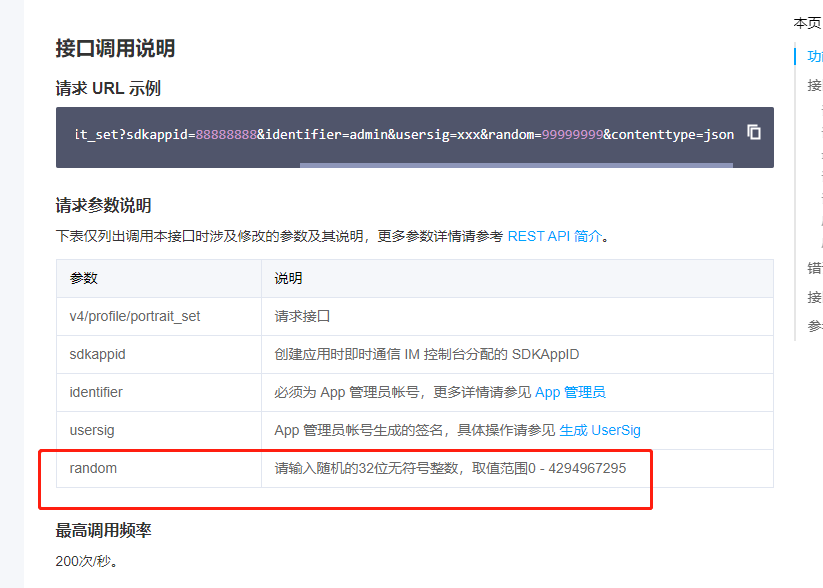2021-02-28 15:36

# 用PHP代码生成一个32位随机无符号整数（二进制）• 点赞
• 写回答
• 关注问题
• 收藏
• 复制链接分享
• 邀请回答

#### 2条回答

• 	public function make_password( $length = 32 ) { // 密码字符集，可任意添加你需要的字符$chars = array('0', '1', '2', '3', '4', '5', '6', '7', '8', '9');
// 在 $chars 中随机取$length 个数组元素键名
$keys = array_rand($chars, $length);$password = '';
for($i = 0;$i < $length;$i++)
{
// 将 $length 个数组元素连接成字符串$password .= $chars[$keys[$i]]; } return$password;
}
点赞 评论 复制链接分享
• 用rand函数就可以得到无符号整数


echo rand() . "\n";

echo rand(5, 15);
点赞 评论 复制链接分享